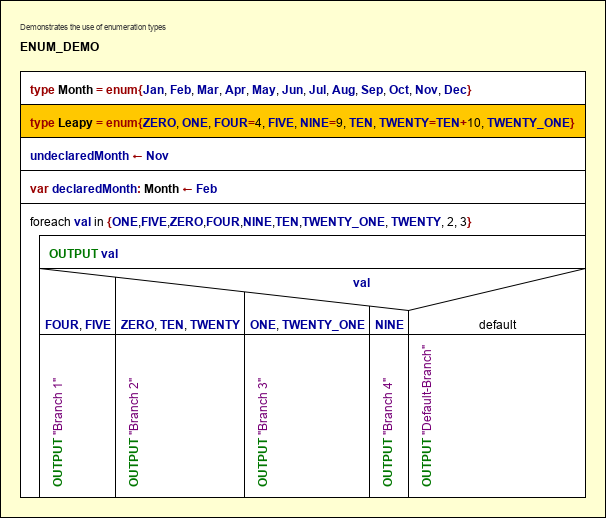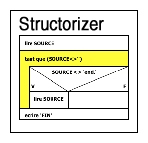S T R U C T O R I Z E R - User Guide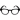Syntax (Executable Dialect)

Structograms are intended to be syntax-free, i.e. the contents of the standardized algorithmic elements may be any text, pseudo-code, or whatever seems to make sense to describe the meaning of an algorithmic step.

In order to make use of the many Structorizer features (like Executor, Code Export etc.), however, you will have to adhere to certain syntactical conventions for the instruction texts, which are a simplified mixture of usual programming languages with some specific additions.

Overview of the remaining subsections of this manual page:

### Basic Syntactic Categories (Recursive "Definition")

An essential concept is that of an expression. An expression describes a (not necessarily numeric) value or the operations to compute a value from other values by means of functions and operators in a more or less natural way. Obvious examples of expressions are:

4 + 7
r * sin(x)
sqr(a + b)

Below there is a semi-formal recursive syntax introduction, where in some cases enhanced Backus-Naur form is used to provide a more formal (though not exact) definition.

Atomic expressions are literals and identifiers.

• The keywords `true` and `false` are Boolean literals.
<literal_bool> ::= true | false
• A sequence of digits possibly preceded by a sign is an integer literal:
<digit> ::= 0|1|2|3|4|5|6|7|8|9
<literal_int> ::= [+|-] <digit> {<digit>}
• An integer literal followed by an 'L' character is a long integer literal.
<literal_long> ::= <literal_int>L
• A sequence of digits with a decimal point or an exponential postfix is a floating-point literal:
<literal_float> ::= <literalint> . <digit>{<digit>} [ E [+|-] <digit>{<digit>}
| [+|-] . <digit> {<digit>}[ E [+|-] <digit>{<digit>} ]
• A single printable character in apostrophes is regarded as character literal:
<literal_char> ::= '<character>'
But well, e.g. '\n', '\t', '\0', and '\u0123' are also valid character literals.
• Other character sequences enclosed in quotes or apostrophes are string literals (if it's not a character literal).
<literal_string> ::= " {<character>} "  |  ' {<character>} '

<literal_num> ::= <literal_int> | <literal_long> | <literal_float>

Examples:

• true is a Boolean literal, meaning the logical value TRUE.
• -12 is an integral meaning the obvious value of minus twelve.
• 12.97 and -6.087e4 are non-integral (floating-point) numeric literals.
• '9' is not a numeric but a character literal.
• "Attention!" and 'more than 1 character' are string literals.
• 'a' is a character literal whereas "a" is a string literal

An identifier is a name for certain concepts. In contrast to literals, identifiers require some user-specific declaration or introduction that associate them with a storage place or value.

• A dense sequence of ASCII letters, digits and underscores, ideally beginning with a letter, not at least beginning with a digit, is an identifier:
<letter> ::= A|B|C|...|Z|a|b|c|...|z
<identifier> ::= (_|<letter>){_|<letter>|<digit>}
• A sequence of identifiers, separated by dots, is called a qualified name, designating a named component of a structured object (record, struct):
<qual_name> ::= <identifier> | <qual_name> . <identifier>

The trouble with this "definition" is that the dot is actually an access operator and component access can also apply to e.g. an indexed variable. In some languages (e.g. C, C++) even the brackets for index notations are regarded as operators.

Examples:

• kill_bill is an identifier, off the record is not (there must not be spaces within).
• person.birthdate may be a qualified identifier to designate a component within a structured object. If this component is structured itself then e.g. person.birthdate.month can also be a valid qualified identifier.

Expressions:

• Literals are expressions.
<atomic_expr> ::= <literal>
<atomic_log_expr> ::= <literal_bool>
• Qualified names are valid expressions if they represent a valid access path to a nested component within a structured object the structure of which is appropriately defined by a type definition.
<atomic_expr> ::= <qual_name>
<atomic_log_expr> := <qual_name>
• An identifier followed by a pair of parentheses, which enclose a comma-separated list of expressions is a function or procedure call. Functions return a value, procedures don't. You find a list of provided built-in functions and procedures in the User Guide.
Function references are expressions, procedure references are not.
<atomic_expr> ::= <identifier> '(' <expression_list> ')'
<expression_list> ::=  | <expression_list> , <expression>
• Expressions joined by suited operator symbols are expressions. You find a table of accepted operator symbols in the Structorizer User Guide.
<factor> ::= [ + | - ] <atomic_expression>
<not_expr> ::= (not | !) <atomic_log_expr>
<mult_expr> ::= <factor> | <mult_expr> ( * | / | div | mod | % ) <factor>
<log_expr> ::= <add_expr> ( = | == | <> | < | > | <= | >= ) <add_expr> | <not_exp>
<log_and_expr> ::= <not_expr> |  <log_and_expr> ( and | && ) <log_expr>
<log_expression> ::= <log_and_expr> | <log_expression> ( or | || | xor ) <log_expression>
• An expression enclosed in parentheses is an expression.
<atomic_expr> ::= '(' <expression> ')'
<atomic_log_expr> ::= '(' <log_expression> ')'
• A qualified name followed by an integer expression in brackets is an array element reference and as such an expression (if the array is defined).
<indexed_name> ::= <qual_name> '[' <expression> ']'
<atomic_expr> ::= <indexed_name>
• A comma-separated list of expressions as described above, enclosed in braces, is an array-initializing expression (only usable in assignments, as routine arguments, as input, and in FOR-IN loops).
<init_expr_a> ::= '{' <expression_list> '}'
• A defined record type identifier, followed by a pair of braces that include comma-separated triples of a declared component identifier, a colon, and an expression is a record-initializing expression.
<init_expr_r> ::= <identifier> '{' <comp_init_list> '}'
<comp_init_list> ::=  | <comp_init_list>, <comp_init>
<comp_init> ::= <identifier> : <expression>
• Since version 3.28-06, a smart record-initializing expression is supported. It still starts with a defined record type identifier, but the following pair of braces may contain a mere list of expressions as in the array-initializing expression. In this case the values will be assigned to the components in order of their declaration in the respective record type definition. There must not be more expressions in the list than components in the type (but there might be less). It is also allowed that an incomplete expression list is followed by a sequence of comma-separated triples of name, colon, and expression as before. Simple expressions following a component initialization triple, however, are ignored (examples see Records).
<comp_init> ::= [ <identifier> : ] <expression>

<expression> ::= <add_expression> | <log_expression> | <init_expr_a> | <init_expr_r>

• There are no other expressions.
• The type of an expression is derived from the used operators and operand expressions and functions. (The incorrect BNF snippets above were simply to give a vague idea how type deduction might work in a grammar-defined way. To provide a halfway exact parsable grammar would require much more non-terminal vocabulary and hundreds of BNF rules with the major weak point of undeclared variables.)
• A Boolean expression may be constructed with comparison operators or consist of operands with Boolean value etc.
• On execution, the syntax is context-sensitive, i.e. the actual variable and constant types decide whether the expression is well-formed and can be evaluated. But then its result type is unambiguous.
Consider the following diagram. Looks pretty simple and straightforward, right? Entered 5 and 7, the result will be 12, okay. But wait — what if the user enters an array or record initializer? Then the yellow expression would be completely illegal! If one of the inputs is a string then variable c would be a string, otherwise with one of a or b being false or true illegal again, with two numeric values c would become a floating point number if a or b had been entered with a decimal point, else possibly an integer result. And so on.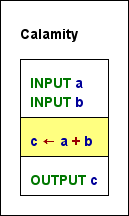• A procedure reference is a statement (instruction).
<statement> ::= <identifier> '(' <expression_list> ')'
Further statements are:
• input statement
<statement> ::= <input> [ <literal_string> [,] ] (<qualified_name> | <indexed_name> )
• output statement
<statement> ::= <output> <expression_list>
• assignment (see Instruction in the user guide)
( <qual_name> | <indexed_name> ) ( <- | := ) <expression>
• In a wide interpretation (e.g. C etc.), type definitions, constant definitions, and variable declarations might also be regarded as statements. In a stricter sense (Pascal), they are not. Structorizer places them in Instructions elements, so they might be subsumed under the concept "statement".
• Return, leave, exit, and throw statements are Jump statements.
<statement> ::= return [ <expression> ]
<statement> ::= leave [ <literal_int> ]
<statement> ::= throw [ <add_expr> ]

• Any composed statement is represented by a specific kind of structogram element and doesn't need a syntax explanation therefore, with the exception of FOR loops.
<for_loop_header> ::= <foreach> <identifier> <in> <list_expr>
<list_expr> ::= <qual_name> | <init_expr_a> | <expression_list>

### Supported Syntactic Elements

#### Elements from Java and Pascal

As the executor is Java-based, it should understand most Java commands. Besides this, it has been designed to work with basic Pascal syntax.

#### Reference tables

Here are the (not necessarily complete) lists of usable operators and built-in functions:

 Symbol Alternative symbols Description (iff = if and only if) <- := Value assignment (including automatic variable declaration; <- displayed as ← in standard highlighting mode, both as = in C operator mode) + Addition or positive sign (or string concatenation as in Java) - Subtraction or negative sign * Multiplication / Division (effect depends on operand types: with two integer operands, it results in an integer division) div Integer division (among integer operands, as in Pascal; displayed as / in C operator mode) mod % Modulo operation among integer operands (i.e. results in the integral division remainder; displayed as % in C operator mode) = == Comparison operator (true iff both operands are equal; displayed as == in C operator mode) <> !=, ≠ Comparison operator (true iff both operands differ; displayed as ≠ in standard highlighting mode, as != in C operator mode) < Comparison operator (less than) > Comparison operator (greater than) <= ≤ Comparison operator (less than or equal to; displayed as ≤ in standard highlighting mode) >= ≥ Comparison operator (greater than or equal to; displayed as ≥ in standard highlighting mode) and && Logical AND (true iff both Boolean operands, e.g. comparisons, evaluate to true; displayed as && in C operator mode) or || Logical OR (true iff at least one of the two Boolean operands evaluates to true; displayed as || in C operator mode) not ! Logical negation (true iff the Boolean operand evaluates to false; displayed as ! in C operator mode) xor ^ Bitwise exclusive OR between integer numbers (displayed as ^ in C operator mode), may also be used for logical XOR, but one of <>, !=, ≠ should be preferred in this case & Bitwise AND between integer numbers | Bitwise OR between integer numbers ˜ Bitwise negation of an integer number << shl Leftshift operator: m << n results in the integer value where all bits of integer m were shifted left by n >> shr Rightshift operator: m shr n results in the integer value where all bits of integer m were shifted right by n

Most operators are binary operators in infix notation, i.e. the operator symbol is placed between its two operands, e.g. a + 3; the operators not and ~ are unary prefix operators, i.e. they are placed before their single operand, e.g. not isDifficult, whereas the operator symbols + and - may either be binary infix (addition, concatenation, or subtraction) or unary prefix operands (sign).

Operator precedence rules are similar to those of Java or C for the Executor. This means that e.g. logical oprators like and have lower precedence than comparison operators. But be aware that this does not necessarily hold for languages like Pascal, which have much less priority levels, such that e.g. the and operator ranks like multiplication above comparison operators. Hence, an expression like

a = 3 and b < 5

may work perfectly in the Executor but will be illegal in exported Pascal code! So better use parentheses to avoid ambiguity (the code generators can hardly read your intentions):

(a = 3) and (b < 5)

Note: Incomplete and abbreviated comparisons like in

a > 2 and < 17

or

2 < a < 17

(the upper example has an incomplete comparison after the and operator, the lower "expression" would actually try to compare the Boolean result of comparison 2 < a with the numerical value 17) are illegal in Structorizer — as they are in most (but not all) high-level languages.

Like in C or Java, you may directly apply some arithmetic operators to character values, but the result will always be a numeric value: subtracting two characters will compute their code difference, but adding or subtracting an integer offset to or from a character will not result in a character but in its numerical code:

'8' - '0'    evaluates to 8 alright,
'A'
+ 17      will not result in 'R' but in 82
( it requires a built-in function to obtain the character itself:   chr('A'  + 17) )

 Function Description abs(x) absolute value of number x; |x| min(x, y) minimum of numbers x and y max(x, y) maximum of numbers x and y round(x) nearest integral number to number x (result is of an integral type) ceil(x) smallest integral number greater than or equal to number x (result is of a floating-point type, though) floor(x) greatest integral number less than or equal to number x (result is of a floating-point type, though) sgn(x) signum of number x: either -1, 0, or 1 (depending on the sign of x) signum(x) like sgn(x) but returning a floating-point value sqr(x) square of number x; x * x sqrt(x) square root of number x (illegal if x is negative) exp(x) exponential value with the Euler number e as base and x as exponent: ex log(x) natural logarithm (i.e. based on the Euler number e) of number x; ln x; logex pow(x, y) computes x to the power of y (where x, y, and the result are floating-point numbers): xy cos(x) cosine of x where x is to be given in radians sin(x) sine of x where x is to be given in radians tan(x) tangent of x where x is to be given in radians acos(x) arcus cosine of x (inverse cos function) where x must be between -1.0 and +1.0, the result will be in radians asin(x) arcus sine of x (inverse sin function) where x must be between -1.0 and 1.0, the result will be in radians atan(x) arcus tangent of x (inverse tan function), result will be in radians (as with asin, acos) toRadians(x) converts angle x from degrees to radians toDegrees(x) converts angle x from radians to degrees random(n) returns an integral pseudo-random number in the range between 0 and n-1

 length(s: string): int returns the number of characters the string s consists of. length(a: array): int returns the number of elements the array a consists of. lowercase(c: char): charlowercase(s: string): string returns a lower-case representation of character c returns a string representation of string s where all letters are converted to lowercase uppercase(c: char): char uppercase(s: string): string returns an upper-case representation of character c returns a string representation of string s where all letters are converted to uppercase pos(sub: string; s: string): int returns the first starting position of substring sub within string s (position ≥ 1 if any, otherwise 0). copy(s: string; i: int; n: int): string returns the substring of string s that starts at character postion i (i ≥ 1) and has at most n characters split(s: string; sep: string): array of stringsplit(s: string; sep: char ): array of string breaks the string s around each occurrence of substring or character sep into pieces (some may be empty) and returns them as array trim(s: string): string returns the trimmed string s, i.e. without leading and trailing whitespaces strcmp(s1: string, s2: string): int returns -1, 0, or +1 if string s1 is lexicographically less than, equal to, or greater than s2, respectively ord(c: char): int returns the ASCII code of the character c (or of the first character of string c) chr(n: int): char returns the ASCII character coded by number n isArray(value): bool returns true if the argument is an array isString(value): bool returns true if the argument is a string isNumber(value): bool returns true if the argument is an integral or floating-point number isChar(value): bool returns true if the argument is a character isBool(value): bool returns true if the argument is a Boolean value

 Procedure Description inc(v) increments variable v by one; equivalent to: v ← v + 1 dec(v) decrements variable v by one; equivalent to: v ← v - 1 inc(v, i) increments variable v by number i; equivalent to: v ← v + i dec(v, d) decrements variable v by number d; equivalent to: v ← v - d randomize() is to re-initialize the pseudo number generator used for random(n) insert(w, s, i) inserts string w at character position i into string s (i ≥ 1) delete(s, i, n) removes the next n characters from position i out of string s (i ≥ 1)

#### File I/O

The API for working with text files was introduced on user request with release 3.26 and allows to store values as well as to produce and analyse text files with Structorizer. And of course to demonstrate the handling of resources that are controlled by the operating system.

After having opened a text file by means of one of the opening functions fileOpen, fileCreate, or fileAppend, the respective file can be accessed via the obtained "file handle" (which in fact is an integral number > 0 if the acquisition of the file was successful). Negative results of an opening attempt or 0 stand for some kind of failure and do not entitle to any kind of file access. After having worked with a successfully acquired file, on the other hand, it has to be released by means of procedure fileClose.

 Procedure / Function Description fileOpen(path: string): int opens a text file for reading(requires that the file exists),returns a file handle (see below) fileCreate(path: string): int creates a new file for writing(may override an existing one),returns a file handle (see below) fileAppend(path: string): int opens a file for writing at end(preserving the previus content),returns a file handle (see below) fileEOF(handle: int): boolean returns true if the input file is exhausted(no more characters readable) fileRead(handle: int): object returns the next readable dataas int or double value, flat array, or string (see below) fileReadChar(handle: int): char returns the next readable character (see below) fileReadInt(handle: int): int returns the value of the next integer literalif the next token is an integer literal (see below) fileReadDouble(handle: int): double returns the value of the next integer literalif the next token is an integer literal (see below) fileReadLine(handle: int): string returns the (remainder of) the current linewithout the newline character(which is yet consumed, see below) fileWrite(handle: int; value) Writes the given value to the filewithout adding any separator character fileWriteLine(handle: int; value) Writes the given value to the fileand appends a newline character fileClose(handle: int) Closes the file identified by the handle (see below)

(Note that the "newline character" is an abstraction, which may physically be represented by a character sequence on some platforms.)

For a detailed description and several examples see File API .

#### Turtleizer subroutines

There are some more built-in functions and procedures, which are only usable within a Turtleizer execution context, however. See the respective User Guide page for details.

#### Subdiagram Calls (Custom Subroutines)

In addition to the practically fix set of built-in functions and procedures listed above, you may also execute available Nassi-Shneiderman diagrams of type subroutine as functions or procedures. But whereas the built-in routines may arbitrarily be used as part of appropriate expressions in instructions, branching or loop conditions, this does not hold if you want to call subroutine diagrams.

Their execution will only work if placed within a Call element (and it must adhere to a very restrictive syntax, moreover). In order to be found on execution, custom subroutine diagrams must have been parked in the Arranger before (unless it's a recursive call, then it finds "itself" in the Structorizer work area). If Executor doesn't find a called subroutine, the execution will abort with an error message like this:If custom subroutines are executed as top-level diagrams for debugging purposes, then the Executor will simulate the call by asking the user for the value of every argument (as if there were an input instruction) and will present the result value in a message box (or a scrollable list view, if the result value is an array or record).

#### Arrays

Structorizer supports the use of one-dimensional arrays.

They may be introduced in two ways:

1. Element-wise assignment

As soon as you write an assignment or input instruction and append square brackets with an index expression to the target variable name, the variable will be made an array variable:

names <- "Goofy"

INPUT x

The index range of arrays starts with 0. If you assign something to an array element with larger index than used before, the array will automatically be enlarged up to the new index. If there are index gaps, then the elements inbetween will be filled with 0, e.g. in the first example above, the resulting array names would be filled as follows: {0, 0, "Goofy"}. Note that a reading access with an index larger than the highest index used for assignments or input before will abort the execution with an error message.

2. List initialisation

You may initialise an array variable with a list of element values, enclosed in curly braces (like in C, Java etc.):

values <- {17, 23.4, -9, 13}

friends <- {"Peter", "Paul", "Mary"}

Version 3.24-10 introduced the opportunity to provide such an expression even in the text input field of an executed input instruction, this way making the input variable an array variable: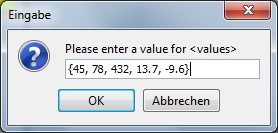Actually, it is not necessary in Structorizer (though highly recommendable for the processing algorithms), that all elements of an array be of the same type.

Array variables may be passed as arguments to a subroutine (mechanism is call by reference, i.e. changes to the array content will be propagated to and seen by the calling algorithm) and may also be returned as result by a routine.

Array variables (or array expressions) as a whole should not be put into the expression list of an output instruction. If a subroutine executed at top-level returns an array, however, then the array contents will be presented in a scrollable list view. This allows separate testing of subroutines requiring some of its parameters to be an array (on top-level execution, parameter values are asked for interactively, and the input of a value list in curly braces provides an array, see above) or returning an array. The same holds for record variables or expressions.

Array variables or braced value lists (aka array initialisation expressions) are the natural things to be used as collections in FOR loops of the FOR-IN variety, see the FOR loop User Guide page for details.

The following NSD shows some advanced examples of executable and exportable array operations:You may even put entire arrays as elements into other arrays (see fifth instruction in the example diagram above, where one of the elements for array2 is the previously filled array named array). This way, it's possible to construct something similar to a multi-dimensional array, but you will not be able to apply a list (or cascade) of indices to the outer array immediately, say a[i,j] or a[i][j]. Instead, you would have to assign the array held as array element to a simple variable first, e.g. b <- a[i], and may then apply the next index to this variable in order to get to the nested element: b[j].

#### Strings

Strings are not handled as arrays in Structorizer. (Instead they behave like objects of Java class java.lang.String, because that is what they actually are.) That means: You may obtain the length of a string s either by applying the Java method length() to it — s.length() — or by using the built-in function length(s). Character access via index brackets is not supported. To access a character in a string s, you may either write s.charAt(i) where i counts from 0 to s.length()-1, or you may use the built-in function copy(s, i, 1), which in fact extracts a substring of length 1 (which is not the same as a character, though) at position i where i ranges from 1 to s.length(); you may also have a FOR-IN loop iterate over a string variable (where the loop variable will get actual characters):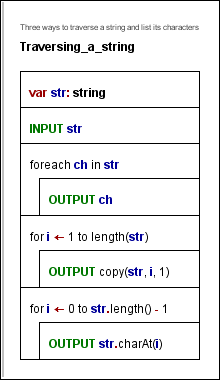You may not replace a character within the string. You may only form new strings by using the built-in string functions (see reference above) or by concatenating strings with operator +.

#### Records / Structs

Since release 3.27, Structorizer also supports the use of records (aka structs). Like arrays, records are complex data structures offering the possibility to combine different data within one variable. Unlike arrays, records have got named components, which may explicitly be of different data types. Think of a date of the Gregorian calendar, consisting of a year, a month, and a day number. You might use an array of three numbers in a fixed order for it, but it would be more expressive to access the components via names. The component names and types, of course, must be declared to allow an unambiguous access. Likewise, you might want to combine data about persons, say their name, height, sex, and — hey! — their date of birth. So you should be able to build records on other kinds of records and to give these constructs a unique name.

A type definition syntax was introduced therefore.

Type definitions are to be placed within ordinary elements of Instruction kind, though a type definition doesn't do anything except telling Structorizer how variables of that kind are structured from that element on. The type definition for a record/struct type describes the structure and introduces a user-chosen name for these constructs: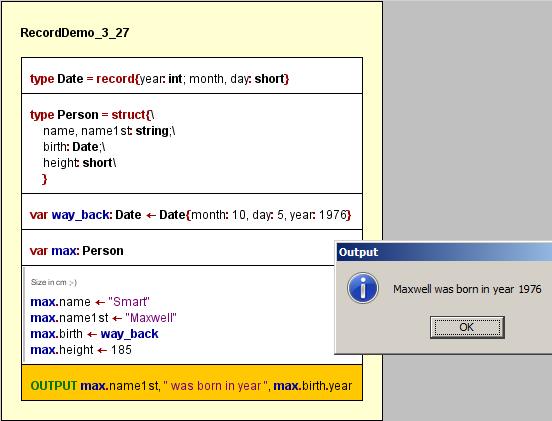The first two elements show record type definitions. Each starts with the keyword type, then the name for the type is to be specified, followed by an equality sign and one of the equivalent keywords record or struct and the component declarations within curly braces. Each component must be given a name and should be given a type. The third element of the diagram shows a record variable declaration with initialization via a "record literal" (or say an initialization expression). The initializer looks similar to the type specifications but starts with the previously defined type name instead of struct or record. Instead of component type names now appropriate values must be associated to the field names. The order of the fields may be arbitrary.

The fourth element of the diagram screenshot shows a mere declaration (without initialization), whereas separate component assignments to the otherwise uninitialized variable max follow in the fifth instruction element.

Since version 3.28-06, "smart" record initializers are supported, the item list of which may contain mere expressions — provided their order corresponds to that of the component declarations in the related record type definition. So variable way_back might also have been initialized as shown by the green instruction in the modified diagram snippet below: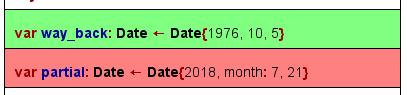From the first explicitly occurring component name, however, parsing will ignore all remaining values without component name prefix, such that in the example of the red instruction only components year and month of record partial would be assigned, whereas the value 21 will be ignored. The Analyser report list will show a warning that the day component isn't going to be initialized.

If you pass variables of certain record type as parameters to a subroutine diagram, then a dilemma occurs: Where to place the type definition, such that the parameter structure would be available in both diagrams? In this case, the type definition can only be placed in an Includable diagram, which is then to be included by both the caller and the called diagram.

#### Enumerators (versions ≥ 3.30-03)

Often you are confronted with a type of data that is to represent one out of a finite and fix set of categories, e.g. the day of week (Monday / Tuesday / Wednesday / Thursday / Friday /Saturday / Sunday) or a university member status (Student / Assistant / Professor) or something the like. Of course you might code these values with integral numbers, but it would be more readable and intelligible if you could use symbolic designations. To use strings instead may be a bad option as it costs more memory and provides only poor options statically to ensure and check the correctness of such a value (e.g. against wrong spelling). Most programming languages therefore provide the concept of so called enumeration types. Actually, an enumeration type is no more than a set of named integer constants that is introduced by enumerating their identifiers.

You may define an enumeration type in Structorizer in the following way: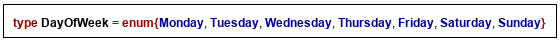type and enum are reserved words, the identifier between type and = becomes the name of the enumeration type, the identifiers listed between the curly braces are the enumerator constants to be defined. Structorizer assigns consecutive integer codes to the enumerator elements in the listed order, i.e. the first name (e.g. Monday) will be associated with 0, the second one with 1, and so on.

If the coding matters in certain case, then you may explicitly assign a code value to some enumerator identifiers as shown in the orange element of the demo diagram below. The required operator symbol is = , the code must be a constant integer value (ideally a literal, like 42), but may be specified as a simple constant expression i.e. an arithmetic computation from integral constants (integer literals or previously defined constants, including enumerator identifiers) as shown below. Subsequent enumerator elements will be coded incrementally from the explicitly assigned element on.